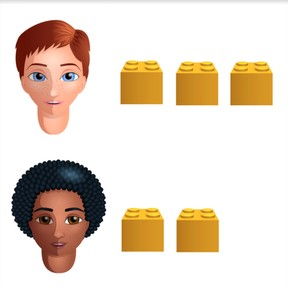Recognizing equal and unequal halves of quantities

# Recognizing equal and unequal halves of quantities

Recognizing equal and unequal halves of amounts8,000 schools use Gynzy92,000 teachers use Gynzy1,600,000 students use Gynzy

## General

Students can recognize equal and unequal halves of amounts to 10. They learn this by dividing different objects and deciding if they are equal halves or not.

K.CC.C

## Relevance

You learn this to know if you can divide something evenly. An example would be if you are going to divide pencils or other objects and want each person to get the same amount.

## Introduction

Students practice recognizing half by indicating which picture shows half of the erasers given in the first image.

## Development

Explain what half is by using the picture of the oranges. If you divide evenly, each person gets half. Next, take six blocks (or other object) and ask two students to come to the front of the class. Ask the students to divide the blocks fairly. How much does the first student have? And the second? Both students have three blocks. They are equal amounts so it has been evenly divided. Take one block away and ask them to divide the blocks again. Is this even? Do they have equal amounts? No, because one has three and the other has two. Five blocks cannot be equally divided between two students. Do the same exercise with the strawberries. Check that students understood by asking them to divide the 11 candies between two students Next ask the students who is right about the division of the cookies.

Check that students have understood by asking the following questions:
When has something been equally divided?
Can you divide something in the classroom into two equal groups?
When is it useful to know if there are equal or unequal halves?

## Guided Practice

Students practice by determining if the shown objects can be equally divided into two groups by choosing the checkmark (yes) or the x (no).

## Closing

Check that students understand by diving the bananas between the two baskets. Can you make equal halves of the bananas? Yes, because each basket gets three bananas. Discuss why it is useful to know if you can equally share an amount. An example would be if each group needs the same amount of pencils.

## Teaching Tip

Allow students who have difficulty with this to practice amounts with a manipulative like blocks, that they can divide into two groups. Student who are ready to be challenged can practice with larger numbers.

## Instruction materials

Blocks or other material that can be divided into groups.

### The online teaching platform for interactive whiteboards and displays in schools

• Save time building lessons

• Manage the classroom more efficiently

• Increase student engagement